20 Mar 2018

# Calculating a Moving Average on Streaming Data

### Moving Average on Streaming Data (4 Part Series)

1. Calculating a Moving Average on Streaming Data

Recently I needed to calculate some statistics (the average and the standard deviation) on a stream of incoming data. I did some research about it, and this article is the result. I’m going to split it into several parts. This first part is about how to calculate the average incrementally. The second part will be about how do the same thing with the standard deviation. A third part will be about the exponential moving average, also known as a low pass filter.

What people often call the ‘average’ is more technically referred to in statistics as the ‘arithmetic mean’. For this article, the terms ‘average’ and ‘mean’ are interchangeable.

The usual way to calculate the average for a set of data that we all learn in school is to add up all of the values (the total), and then divide by the number of values (the count):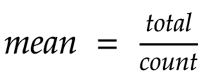Here’s the math notation that describes what I just wrote above: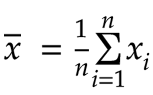Below is a simple javascript function that uses this naive approach to obtain the mean:

``````const simpleMean = values => {
validate(values)

const sum = values.reduce((a,b)=>a+b, 0)
const mean = sum/values.length
return mean
}

const validate = values =>  {
if (!values || values.length == 0) {
throw new Error('Mean is undefined')
}
}
``````

While this logic is fine as far as it goes, it does have a couple of limitations in practice:

• We accumulate a potentially large sum, which can cause precision and overflow problems when using floating point types.
• We need to have all of the data available before we can do the calculation.

Both of these problems can be solved with an incremental approach where we adjust the average for each new value that comes along. I’ll show how to derive this formula with some math first, and then I’ll show a JavaScript implementation.

There are two symbols in math that are often used to denote the mean. `σ`, the lowercase greek letter sigma, refers to the population mean. This is the average for an entire population - all of the possible values. The average of all the grades on a particular test is an example.

`x̄`, pronounced x-bar, refers to the sample mean. This is the average of a sample of values from the total population. You might take a random sample of people across the country to find the average height of the population, but it’s impractical to measure the height of every single person in an entire country. Of course when using a sample, it’s desirable to try to get as close as possible to the mean of the population the sample represents.

I decided to use the sample notation for this article to indicate that the average we’re calculating could be based on a sample.

Okay, let’s start with the formula for the average that we saw earlier:Let’s split up the sum so that we add up the first n-1 values first, and then we add the last value xn.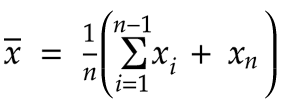We know that the average = total / count:Let’s rearrange this a bit: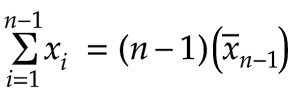Here’s the result of applying the above substitution to the total of the first n-1 values:Let’s expand this:Rearranging a bit, we get: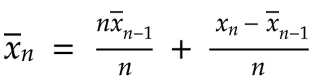We can cancel out the `n`’s in the first fraction to obtain our final result: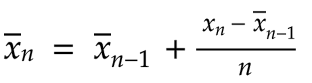What does this all really mean? We now have a recurrence relation that defines our mean for the nth value as follows: Add a differential to whatever the mean was for the previous n-1 values. Each time we add a new value, all we have to do is to calculate this differential and add it to the previous average. This now becomes the new average.

Below is a simple implementation of this idea:

``````class MovingAverageCalculator {
constructor() {
this.count = 0
this._mean = 0
}

update(newValue) {
this.count++

const differential = (newValue - this._mean) / this.count

const newMean = this._mean + differential

this._mean = newMean
}

get mean() {
this.validate()
return this._mean
}

validate() {
if (this.count == 0) {
throw new Error('Mean is undefined')
}
}
}
``````

In the above code, each time we call `update` with a new value, we increment the count and calculate our differential. `newMean` is the previous average added to this differential. That now becomes the average that will be used the next time we call `update`.

Below is a simple comparison of the two methods:

``````console.log('simple mean = ' + simpleMean([1,2,3]))

const calc = new MovingAverageCalculator()
calc.update(1)
calc.update(2)
calc.update(3)
console.log('moving average mean = ' + calc.mean)
``````

The result is as expected:

``````C:\dev\>node RunningMean.js
simple mean = 2
moving average mean = 2
``````

There are of course many other kinds of moving averages that are possible, but if you simply want a cumulative moving average, this logic works well: It’s simple, you can apply it to a streaming data set, and it sidesteps problems with precision and overflow that can happen with the naive approach.

Before concluding, I’d like to derive one more identity using our last result. We don’t need it right now, but we’ll use in the next article.Let’s subtract the first term on the right from both sides, giving us the value of just our differential: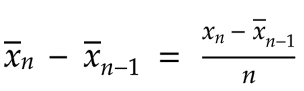Now let’s multiply by n: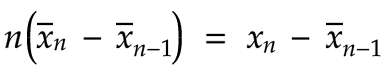Let’s multiply both sides by -1: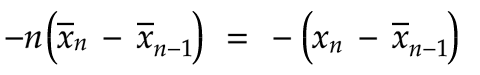And finally let’s mutiply the -1 through both sides: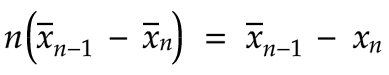We’ll just hold on to this identity for now, but it will be useful in part 2 where we derive the formula for incrementally calculating the variance and standard deviation.

Related: